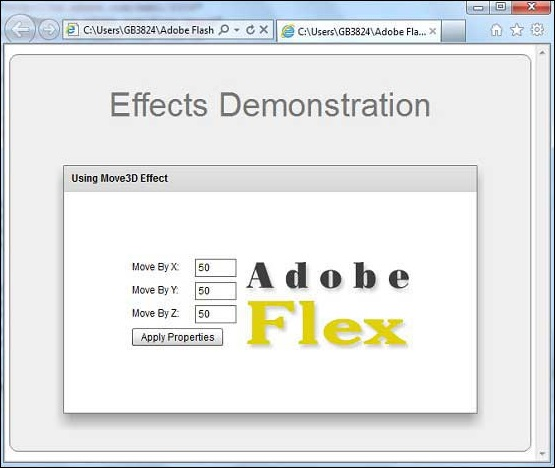# Flex - Move3D Effect

## Introduction

The Move3D class moves a target object in the x, y, and z dimensions. The x, y, and z property specifications of the Move3D effect specify the change in x, y, and z that should occur to the transform center around which the overall transform effect occurs.

## Class Declaration

Following is the declaration for spark.effects.Move3D class −

```public class Move3D
extends AnimateTransform3D
```

## Public Properties

Sr.No Property & Description
1

xBy : Number

Number of pixels by which to modify the x position of the target.

2

xFrom : Number

Initial x position of the target, in pixels.

3

xTo : Number

Final x, in pixels.

4

yBy : Number

Number of pixels by which to modify the y position of the target.

5

yFrom : Number

Initial y position of the target, in pixels.

6

yTo : Number

Final y position of the target, in pixels.

7

zBy : Number

Number of pixels by which to modify the z position of the target.

8

zFrom : Number

Initial z position of the target.

9

zTo : Number

Final z position of the target.

## Public Methods

Sr.No Method & Description
1

Move3D(target:Object = null)

Constructor.

## Methods Inherited

This class inherits methods from the following classes −

• spark.effects.AnimateTransform3D
• spark.effects.AnimateTransform
• spark.effects.Animate
• mx.effects.Effect
• flash.events.EventDispatcher
• Object

## Flex Move3D Effect Example

Let us follow the following steps to check usage of Move3D Effect in a Flex application by creating a test application −

Step Description
1 Create a project with a name HelloWorld under a package com.tutorialspoint.client as explained in the Flex - Create Application chapter.
2 Modify HelloWorld.mxml as explained below. Keep rest of the files unchanged.
3 Compile and run the application to make sure business logic is working as per the requirements.

Following is the content of the modified mxml file src/com.tutorialspoint/HelloWorld.mxml.

```<?xml version = "1.0" encoding = "utf-8"?>
width = "100%" height = "100%" minWidth = "500" minHeight = "500">

<fx:Style source = "/com/tutorialspoint/client/Style.css" />
<fx:Script>
<![CDATA[
private function applyMoveProperties():void {
moveEffect.play();
}
]]>
</fx:Script>

<fx:Declarations>
<s:Move3D id = "moveEffect" target = "{imageFlex}"
xFrom = "{imageFlex.x}" xBy = "{Number(moveX.text)}"
yFrom = "{imageFlex.y}" yBy = "{Number(moveY.text)}"
zFrom = "{imageFlex.z}" zBy = "{Number(moveZ.text)}" />
</fx:Declarations>

<s:BorderContainer width = "630" height = "480" id = "mainContainer"
styleName = "container">
<s:VGroup width = "100%" height = "100%" gap = "50"
horizontalAlign = "center" verticalAlign = "middle">
<s:Label id = "lblHeader" text = "Effects Demonstration"
fontSize = "40" color = "0x777777" styleName = "heading" />

<s:Panel id = "move3DPanel" title = "Using Move3D Effect"
width = "500" height = "300">
<s:layout>
<s:HorizontalLayout  gap = "10" verticalAlign = "middle"
horizontalAlign = "center" />
</s:layout>

<s:VGroup top = "10" left = "15">
<s:HGroup verticalAlign = "middle">
<s:Label text = "Move By X:" width = "70" />
<s:TextInput id = "moveX" text = "50" width = "50" />
</s:HGroup>

<s:HGroup verticalAlign = "middle">
<s:Label text = "Move By Y:" width = "70" />
<s:TextInput id = "moveY" text = "50" width = "50" />
</s:HGroup>

<s:HGroup verticalAlign = "middle">
<s:Label text = "Move By Z:" width = "70" />
<s:TextInput id = "moveZ" text = "50" width = "50" />
</s:HGroup>

<s:Button label = "Apply Properties" click = "applyMoveProperties()" />
</s:VGroup>

<s:Image id = "imageFlex"
source = "http://www.tutorialspoint.com/images/flex-mini.png" />
</s:Panel>
</s:VGroup>
</s:BorderContainer>
</s:Application>
```

Once you are ready with all the changes done, let us compile and run the application in normal mode as we did in Flex - Create Application chapter. If everything is fine with your application, it will produce the following result: [ Try it online ]flex_visual_effects.htm